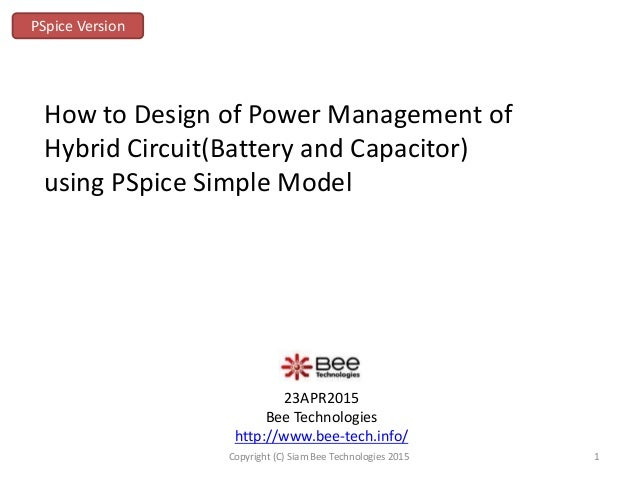Successfully reported this slideshow.
We use your LinkedIn profile and activity data to personalize ads and to show you more relevant ads. You can change your ad preferences anytime.Upcoming SlideShare
Loading in …5
×

# How to Design of Power Management of Hybrid Circuit(Battery and Capacitor) using PSpice Simple Model

36,083 views

Published on

How to Design of Power Management of Hybrid Circuit(Battery and Capacitor) using PSpice Simple Model

Published in: Engineering
• Full Name
Comment goes here.

Are you sure you want to Yes No
Your message goes here• Be the first to comment

### How to Design of Power Management of Hybrid Circuit(Battery and Capacitor) using PSpice Simple Model

1. 1. 23APR2015 Bee Technologies http://www.bee-tech.info/ How to Design of Power Management of Hybrid Circuit(Battery and Capacitor) using PSpice Simple Model PSpice Version 1Copyright (C) Siam Bee Technologies 2015
2. 2. 1.Circuit Method 2.Battery System only 3.Battery and Capacitor System 4.Conclusion PSpice Version SPICE Model using Simple Model by Bee Technologies Lithium Ion Battery Model http://www.bee-tech.info/simple-model-for-spice/lithium-ion-battery-model/ Lithium Ion Capacitor Model http://www.bee-tech.info/simple-model-for-spice/lithium-ion-capacitor-model/ 2Copyright (C) Siam Bee Technologies 2015
3. 3. 1.Circuit Method Battery System only Hybrid(Battery and Capacitor) System Lithium Ion Battery Current Load Lithium Ion Battery Current Load Lithium Ion Capacitor Control Current Load Profile ILOAD T1 = 0 T2 = 25s I1 = 0 I2 = {Max_Load} I3 = {Min_Load} I4 = {Min_Load} I5 = {Max_Load} I6 = {Min_Load} T3 = 50s T4 = 120s T5 = 150s T6 = 180s 0 0 Load PARAMETERS: Max_Load = 11 Min_Load = 2 Hybrid(Battery and Capacitor) System If Current load >4[A], Lithium Ion Capacitor work If Current load =<4[A], Lithium Ion Battery work 3Copyright (C) Siam Bee Technologies 2015
4. 4. 1.Circuit Method Current Load Profile Hybrid(Battery and Capacitor) System If Current load >4[A], Lithium Ion Capacitor work If Current load =<4[A], Lithium Ion Battery work Lithium Ion Capacitor work Lithium Ion Battery work 4Copyright (C) Siam Bee Technologies 2015
5. 5. 2.Battery System only IN- OUT+ OUT- IN+ G1 LIMIT(V(%IN+, %IN-)/10u, 0 , I(Load)) ILOAD T1 = 0 T2 = 25s I1 = 0 I2 = {Max_Load} I3 = {Min_Load} I4 = {Min_Load} I5 = {Max_Load} I6 = {Min_Load} T3 = 50s T4 = 120s T5 = 150s T6 = 180s 0 0 Load PARAMETERS: Max_Load = 11 Min_Load = 2 0 VBAT 0 DIS_BAT + - U1 LI-ION_BATTERY SOC = 1 NS = 1 TSCALE = 1 C = 2 5Copyright (C) Siam Bee Technologies 2015
6. 6. 2.Battery System only Current Load Battery Voltage Battery SOC(%): 100->83.472 6Copyright (C) Siam Bee Technologies 2015
7. 7. 3.Battery and Capacitor System PARAMETERS: Dis_SW = 4A 0 ILOAD T1 = 0 T2 = 25s I1 = 0 I2 = {Max_Load} I3 = {Min_Load} I4 = {Min_Load} I5 = {Max_Load} I6 = {Min_Load} T3 = 50s T4 = 120s T5 = 150s T6 = 180s 0 DIS_BAT IN+ IN- OUT+ OUT- E1 IF(I(Load)>Dis_SW, 0, 1) 0 0 00 Ctrl_BAT PARAMETERS: Max_Load = 11 Min_Load = 2 Load IN- OUT+ OUT- IN+ G1 LIMIT(V(Ctrl_BAT, 0)/10u, 0, I(Load)) + - + - S1 VON = 0.5 VOFF = 0.3 ROFF = 100e6 RON = 100u 0 0 + - U1 LI-ION_BATTERY SOC = 1 NS = 1 TSCALE = 1 C = 2 0 VCAP 0 DIS_CAP IN+ IN- OUT+ OUT- E2 IF(I(Load)>Dis_SW, 1, 0) 0 Ctrl_CAP 0 IN- OUT+ OUT- IN+ G2 LIMIT(V(Ctrl_CAP, 0)/10u, 0, I(Load)) + - + - S2 VON = 0.5 VOFF = 0.3 ROFF = 100e6 RON = 100u 0 0 VBAT U2 LI-ION_CAPACITOR CAP = 500 ESR = 0.8m RDC = 1.2m NS = 1 TSCAL = 1 SOC = 100 7Copyright (C) Siam Bee Technologies 2015
8. 8. Control Battery Voltage Battery SOC(%): 100->92.012 3.Battery and Capacitor System Current Load Capacitor Voltage Capacitor SOC(%): 100->21.714 8Copyright (C) Siam Bee Technologies 2015
9. 9. 4. Conclusion Battery System only Hybrid(Battery and Capacitor) System Lithium Ion Battery Current Load Lithium Ion Battery Current Load Lithium Ion Capacitor Control SOC[%] Start Stop Lithium Ion Battery 100 83.472 SOC[%] Start Stop Lithium Ion Battery 100 92.012 Lithium Ion Capacitor 100 21.714 If high Current wave form quickly, Hybrid Circuit is better. 9Copyright (C) Siam Bee Technologies 2015